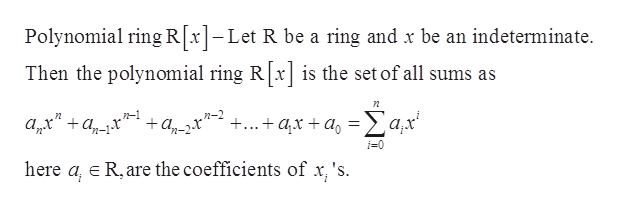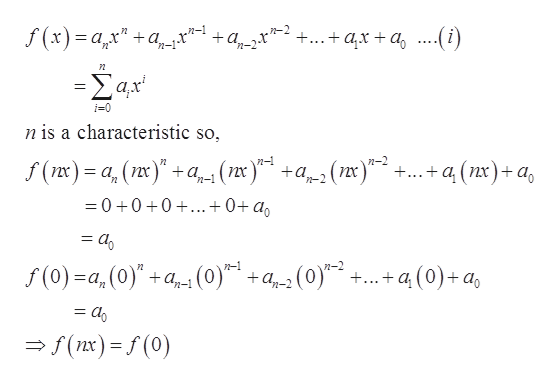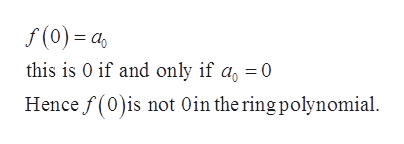# In a ring, the characteristic is the smallest integer n such that nx=0 for all x in the ring.  Is it acceptable to take "f" of both sides to get:f(nx)=f(0) in the corresponding polynomial ring?If so, is f(0) 0 in the polynomial ring?And can we write f(nx) as nf(x)?

Question
50 views

In a ring, the characteristic is the smallest integer n such that nx=0 for all x in the ring.  Is it acceptable to take "f" of both sides to get:

f(nx)=f(0) in the corresponding polynomial ring?

If so, is f(0) 0 in the polynomial ring?

And can we write f(nx) as nf(x)?

check_circle

star
star
star
star
star
1 Rating
Step 1

Definition of Polynomial ring-help_outlineImage TranscriptionclosePolynomial ring R[x1-Let R be a ring and x be an indeterminate Then the polynomial ring Rx is the set of all sums as Σαχ +...axa а,х" + а,-х i=0 here a, E R, are the coefficients of x 's fullscreen
Step 2

Yes, f(nx)=f(0)

In a ring, the characteristic is the smallest integer n such that nx=0 for all x in the ring.help_outlineImage Transcriptionclosef(x)aa +...+ax +a....(i) +a, -Σαx. i-0 n is a characteristic so, n-2 2 f(x)= a (x + (x)a(x) +..+a (nx)+ a =0 +0 0 ...+0+ a = a0 f(0)-a,(0)"+a (0) n-1 +а,-2 +...+a (0)a = 40 f(nx)(0) fullscreen
Step 3

From the ab...help_outlineImage Transcriptionclosef(0)= as this is 0 if and only if a = 0 Hence f(0)is not 0in the ring polynomial fullscreen

### Want to see the full answer?

See Solution

#### Want to see this answer and more?

Solutions are written by subject experts who are available 24/7. Questions are typically answered within 1 hour.*

See Solution
*Response times may vary by subject and question.
Tagged in

### Math